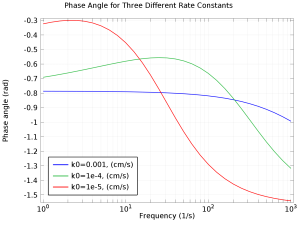# アプリケーションギャラリ

## Electrochemical Impedance Spectroscopy

Application ID: 14431

The purpose of this app is to understand EIS, Nyquist, and Bode plots. The app lets you vary the bulk concentration, diffusion coefficient, exchange current density, double layer capacitance, and the maximum and minimum frequency.

Electrochemical impedance spectroscopy (EIS) is a common technique in electroanalysis used to study the harmonic response of an electrochemical system. A small, sinusoidal variation is applied to the potential at the working electrode, and the resulting current is analyzed in the frequency domain.

The real and imaginary components of the impedance give information about the kinetic and mass transport properties of the cell, as well as the surface properties through the double layer capacitance.This application example illustrates applications of this type that would nominally be built using the following products: